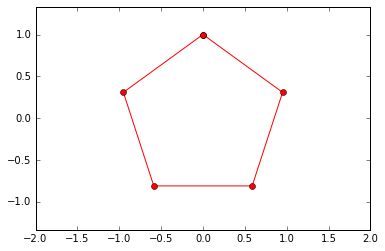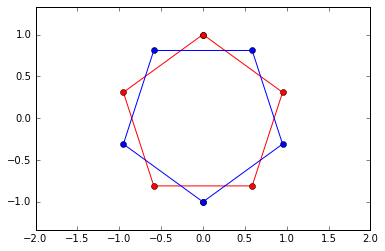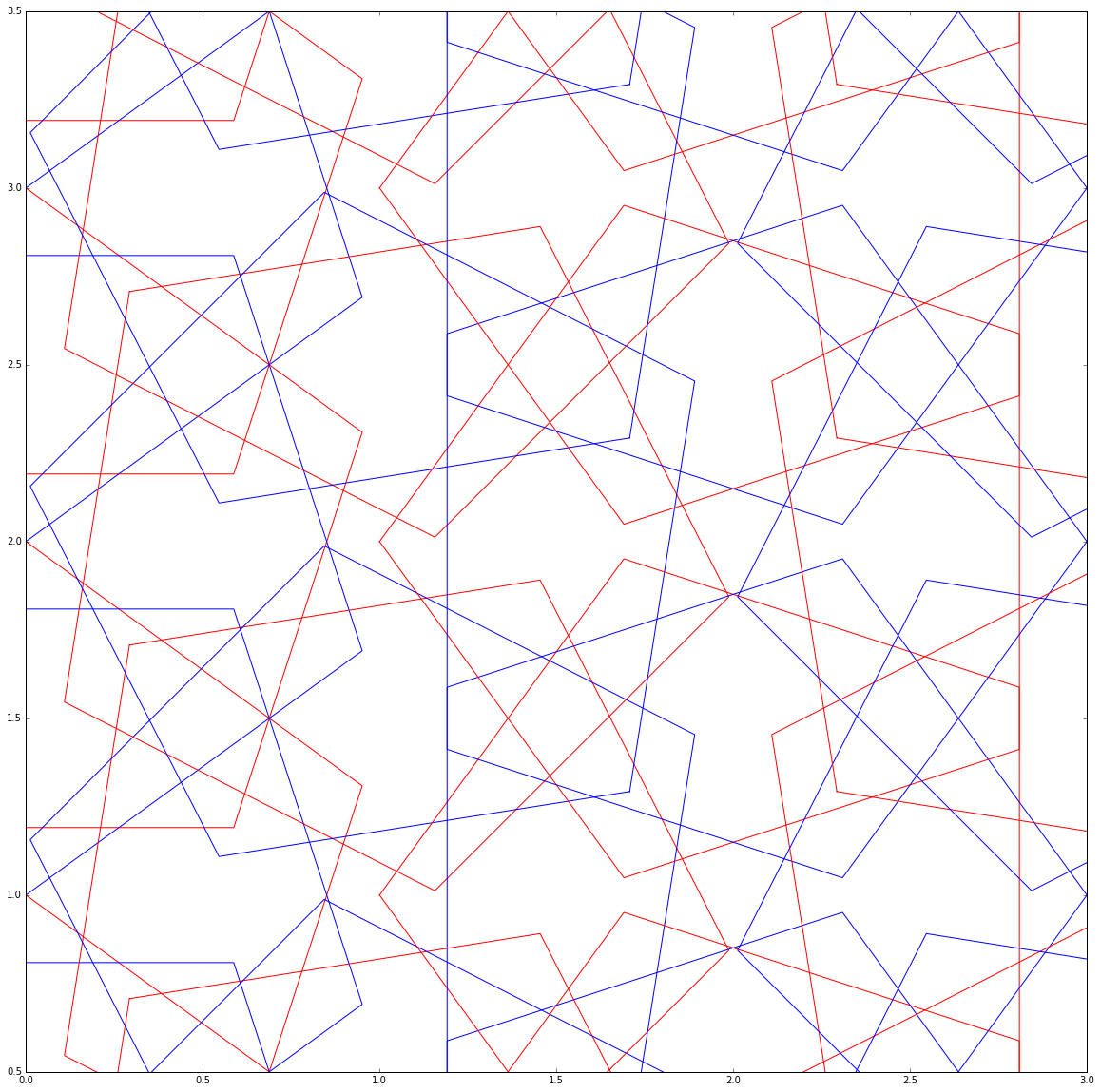# Linear Algebra III - Primitive Shapes in NumpyAl Jabr pattern, created using numpy and matplotlib © Sam Swift-Glasman

In this post we will start to break down techniques used to generate the piece Al-Jabr. This can be found on the first post in this series. In the embedded IPython notebook below, you will find an implementation of geometric arabic design using the numpy and matplotlib. These libraries as well as another called pandas, are the bread and butter of scientific python so this is a great chance to show some possibilities.

The Jupyter Notebook can be found with all of the other source files on this website at the Learning Machine Learning Github. I recommend you clone the repo and have a play with some of the parameters to get some different patterns.

https://github.com/glassman3d/learningmachinelearning.git

A few notes about the maths and code below:

-The vectors and matrices are all in augmented matrix form to allow composition of transformations. Therefore 2D vectors are represented as 3d vectors, these are called homogenous coordinates.
More info about this can be found here and here.

-Numpy and Matplotlib are worth learning, there are some interesting examples below of numpy array slicing and multiple matplotlib figures. Lots of great tutorials out there for the two, will do at least a whole post on each library later in the series.

I hope you enjoy this as much as i did, comments below if you have any questions - next post will be implementing this in Houdini and Vex.

In :
```%matplotlib inline
import matplotlib.pyplot as plt
import numpy as np
```
In :
```#starting parameters
num_sides = 5
init_scale = 1

"""A single point has the following in initial attributes in our 2D model:
Note that we are using 3d vectors to represent our 2d coords (homogeneous coords)
These will allow us to use square matrices to compose transformations"""

# Position at the origin (0,0)
single_P = np.array([0,0,1])
# An initial normal pointing along the y axis)
single_N = np.array([0,1,1])

print "P: {0}".format(single_P)
print "N: {0}".format(single_N)
```
```P: [0 0 1]
N: [0 1 1]
```
In :
```"""next up we will make an array of point normals, one for each vertex of our shape"""

shape_pts_N = []

for i in range(num_sides+1):
theta = i * np.radians(float(360)/num_sides)
c,s = np.cos(theta), np.sin(theta)
R = np.matrix([[c,s,0],[-s,c,0],[0,0,1]])
vec = single_N *init_scale * R
shape_pts_N.append(vec)

"""We will use this array to create a matrix of points, one for each vertex of our shape"""

shape_pts_P = np.array(shape_pts_N).reshape(num_sides+1,3)
print shape_pts_P
```
```[[  0.00000000e+00   1.00000000e+00   1.00000000e+00]
[ -9.51056516e-01   3.09016994e-01   1.00000000e+00]
[ -5.87785252e-01  -8.09016994e-01   1.00000000e+00]
[  5.87785252e-01  -8.09016994e-01   1.00000000e+00]
[  9.51056516e-01   3.09016994e-01   1.00000000e+00]
[  2.44929360e-16   1.00000000e+00   1.00000000e+00]]
```
In :
```"""Now we can plot  our basic shape"""
plt.plot(shape_pts_P[:,0], shape_pts_P[:,1],'ro-')
plt.axis('equal')
plt.axis([-2,2,-2,2])
plt.show()
```In :
```"""We duplicate and flip the shape"""
theta = np.radians(180)
c,s = np.cos(theta), np.sin(theta)
R = np.matrix([[c,s,0],[-s,c,0],[0,0,1]])
shape_pts_flip = shape_pts_P * R
print "shape_pts_P:\n", shape_pts_P,"\n"
print "shape_pts_flip:\n", shape_pts_flip

plt.figure(1)
plt.plot(shape_pts_P[:,0], shape_pts_P[:,1],'ro-')
plt.plot(shape_pts_flip[:,0], shape_pts_flip[:,1],'bo-')
plt.axis('equal')
plt.axis([-2,2,-2,2])
plt.show()
```
```shape_pts_P:
[[  0.00000000e+00   1.00000000e+00   1.00000000e+00]
[ -9.51056516e-01   3.09016994e-01   1.00000000e+00]
[ -5.87785252e-01  -8.09016994e-01   1.00000000e+00]
[  5.87785252e-01  -8.09016994e-01   1.00000000e+00]
[  9.51056516e-01   3.09016994e-01   1.00000000e+00]
[  2.44929360e-16   1.00000000e+00   1.00000000e+00]]

shape_pts_flip:
[[ -1.22464680e-16  -1.00000000e+00   1.00000000e+00]
[  9.51056516e-01  -3.09016994e-01   1.00000000e+00]
[  5.87785252e-01   8.09016994e-01   1.00000000e+00]
[ -5.87785252e-01   8.09016994e-01   1.00000000e+00]
[ -9.51056516e-01  -3.09016994e-01   1.00000000e+00]
[ -3.67394040e-16  -1.00000000e+00   1.00000000e+00]]
```In :
```"""Now we can go a bit mad and add some looping to create a pattern
We'll be composing matrix and translations into one matrix"""

plt.figure(1,figsize=(20, 20))
tY = 1
tX = 1
iterations = 6

for i in range(iterations):
for j in range(iterations):
#theta = i * np.radians(float(360)/iterations)
theta = i*np.radians(45)
c,s = np.cos(theta), np.sin(theta)
R = np.matrix([[c,s,0],[-s,c,0],[0,0,1]])
T = np.matrix([[1,0,0],[0,1,0],[tY*i,tX*j,1]])
C = R*T
sP = shape_pts_P*C
sF = shape_pts_flip*C
plt.plot(sP[:,0], sP[:,1],'r-')
plt.plot(sF[:,0], sF[:,1],'b-')
plt.axis('equal')
plt.axis([0,iterations/2,1,iterations/2])
plt.show()
```